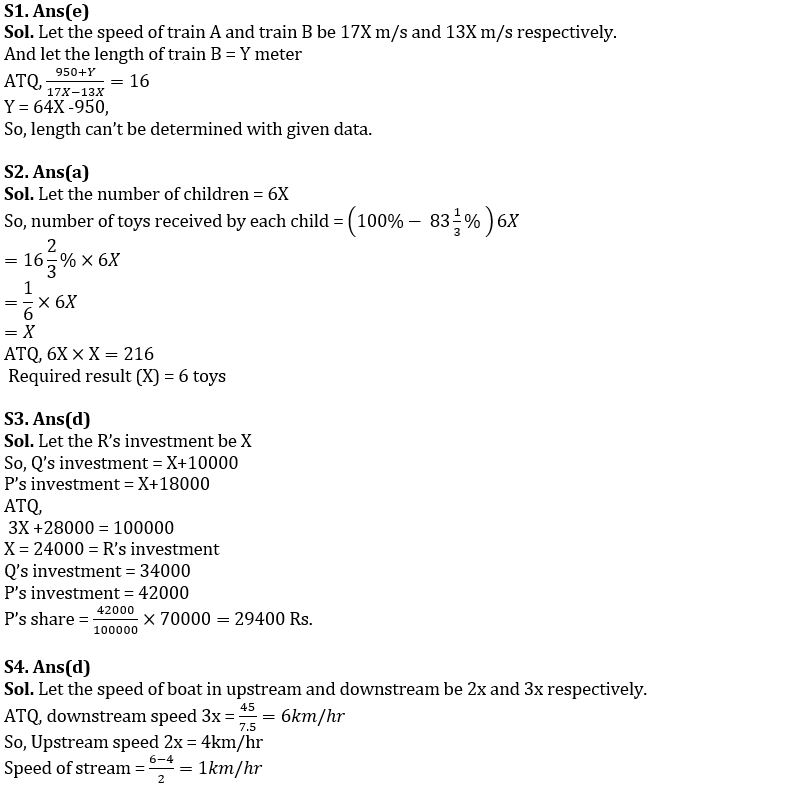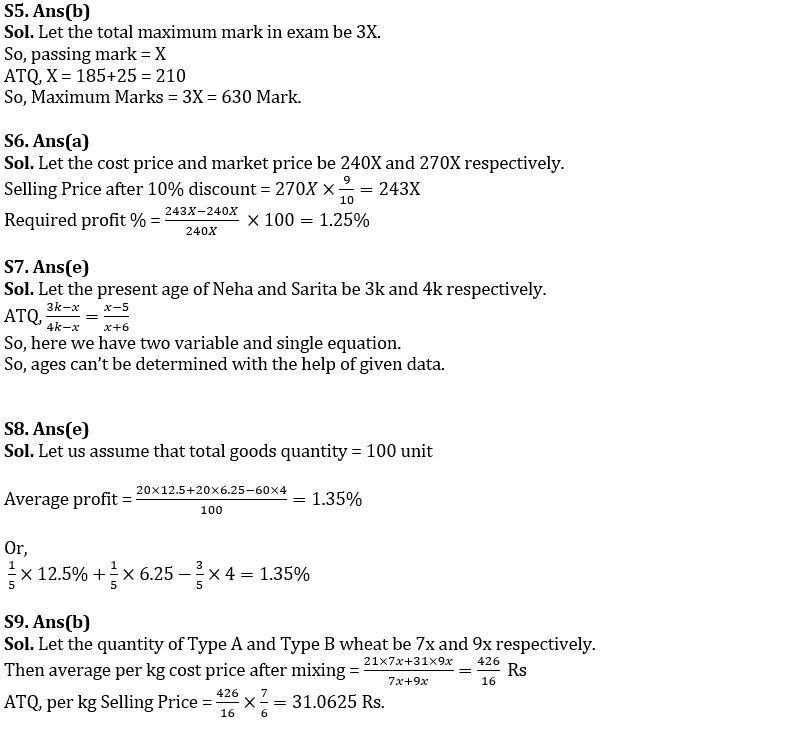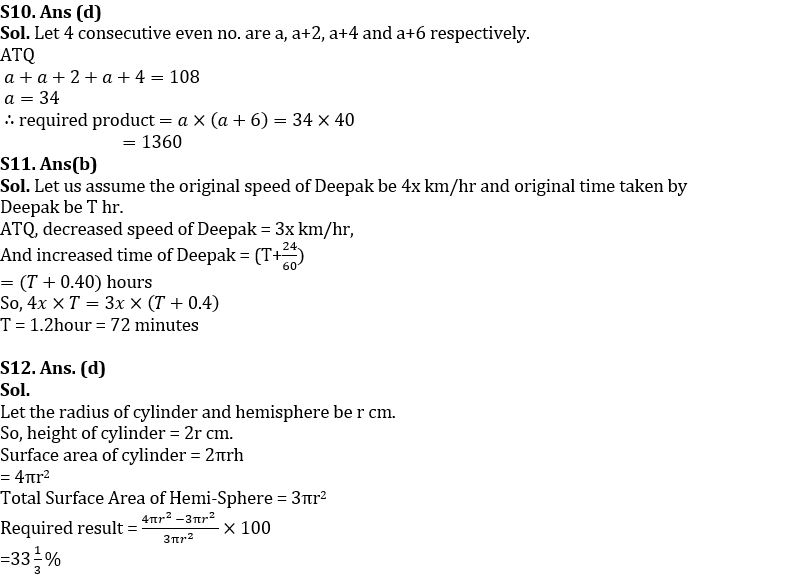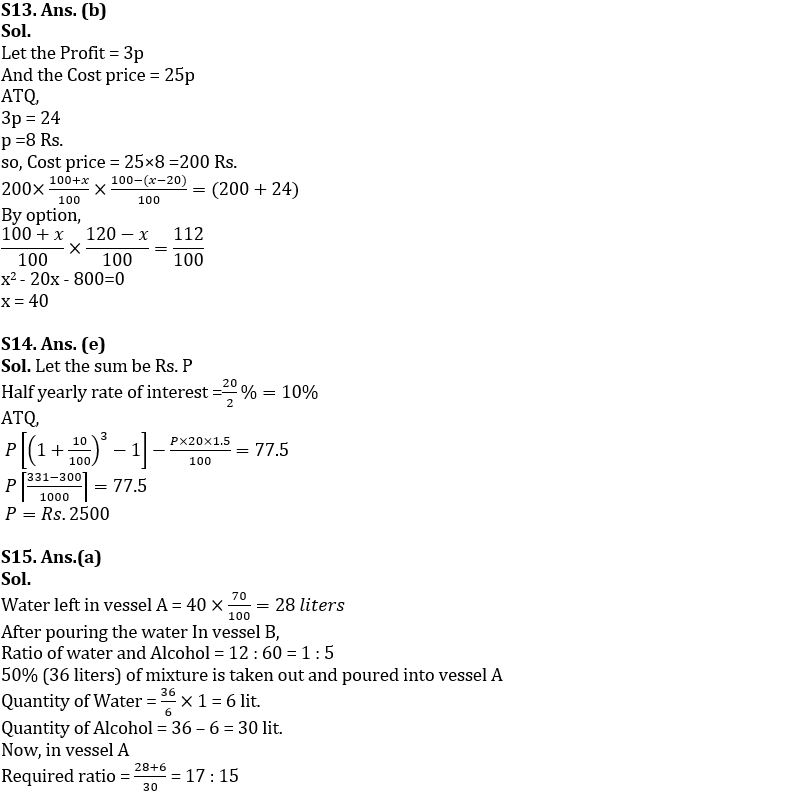Latest Banking jobs   »

# Quantitative Aptitude Quiz For Bank Foundation 2023- 23rd January

Q1. A 950 metres long train-A crosses another train-B running in same direction in 16 seconds. If the ratio of speed of these trains is in the ratio 17:13 respectively, find out the length of train B?
(a) 1000 meter
(b) 1900 meter
(c) 1600 meter
(d) 1100 meter
(e) Can’t be determined

Q2. If 216 toys were distributed equally among children in such a way that the number of toys received by each child is 83⅓% less than the total number of children. How many toys did each child receive?
(a) 6 toys
(b) 12 toys
(c) 9 toys
(d) 36 toys
(e) 27 toys

Q3. P, Q, R together invested Rs. 100000 in a business. if P invested Rs. 8000 more than Q and Q invested Rs. 10000 more than R, then at the end of the year what will be share of P out of a total profit of Rs. 70,000?
(a) 30600 Rs.
(b) 28000 Rs.
(c) 27500 Rs.
(d) 29400 Rs.
(e) 30000 Rs.

Q4. Find the speed of stream, if a boat covers 45 km in downstream in 7 ½ hours and ratio of upstream and downstream speed of boat is 2:3 respectively.
(a) 2km/hr
(b) 1.5km/hr
(c) 3km/hr
(d) 1km/hr
(e) 3.5km/hr

Q5. A student has to obtain 33⅓% of the total marks to pass an examination. He got 185 marks and failed by 25 marks. The maximum marks for the examination are?
(a) 660
(b) 630
(c) 570
(d) 540
(e) 510

Q6. The ratio of cost price and market price of an article is 24:27. If 10% discount is given for article, then find the profit percentage.
(a) 1.25%
(b) 1.75%
(c) 1.50%
(d) 2.50%
(e) 2.25%

Q7. Ratio of Neha and Sarita’s age is 3:4, x years ago the ratio was (x-5) :(x+6). Find out present age of Sarita. (in years)
(a) 16
(b) 24
(c) 32
(d) 12
(e) Can’t be determined.

Q8. A shopkeeper sells one-fifth of his goods at a profit of 12.5%, another one-fifth at a profit of 6 ¼ %, and the rest at a loss of 4%. What is his overall profit percentage?
(a) 1.75%
(b) 1.65%
(c) 1.25%
(d) 1.55%
(e) 1.35%

Q9. The cost price of Type A wheat is Rs. 21 per kg and Type B wheat is Rs.31 per kg. If both Type A and Type B wheat are mixed in the ratio of 7: 9 respectively, and the resultant mixture is sold at 16⅔ % profit then find out the per kg selling price of the mixture.
(a) 30.0625 Rs.
(b) 31.0625 Rs.
(c) 32.0225 Rs.
(d) 33.0225 Rs.
(e) 34 Rs.

Q10. There are 4 consecutive even numbers. If sum of first three numbers is 108, then calculate the product of smallest and largest no.
1260
1292
1280
1360
1428

Q11. Deepak takes 24 minutes more to cover a certain distance by decreasing his speed by 25%. What is the time taken by him to cover the distance with his original speed?
(a) 70 minutes
(b) 72 minutes
(c) 75 minutes
(d) 90 minutes
(e) 84 minutes

Q12. The ratio of height of a cylinder to its base radius is 2:1 respectively. If radius of a hemisphere is equal to the radius of the cylinder, then find the total surface area of cylinder is what percent more than total surface area of a hemisphere?
(a) 40%
(b) 30%
(c) can’t be determined
(d) 33⅓%
(e) 50%

Q13. A shopkeeper marked up price of an article by x% above its cost price and offers a discount of (x-20) % on it, so that the selling price becomes Rs. 24 more than its cost price. If ratio of cost price to profit is of 25:3, then find the value of ‘x’.
(a) 25
(b) 40
(c) 20
(d) 50
(e) 100

Q14. Compound interest on a certain sum at the rate of 20% payable half yearly for 1.5 years is Rs.’77.5’ more than the Simple interest for 1.5 years on same sum at the same rate (p.a.). Find the Sum?
(a) Rs. 2600
(b) Rs. 2200
(c) Rs. 2700
(d) Rs. 2300
(e) Rs. 2500

Q15. Two vessels A and B containing 40 lit of water and 60 lit of alcohol respectively. If 30% of water is taken from vessel A and poured into vessel B and again 50% mixture is taken out from Vessel B and poured into Vessel A, then find the ratio between water to alcohol in final mixture in vessel A .
(a) 17 : 15
(b) 15 : 13
(c) 3 : 2
(d) 4 : 3
(e) 16 : 15

Solutions## FAQs

### When is the IBPS SO Mains exam scheduled to be held?

The IBPS SO Mains exam is scheduled to be held on 29th January 2023.

#### Congratulations!